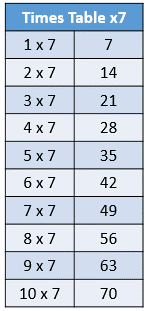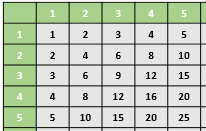# How to Learn Times Tables

Instructor: Heather Jenkins

Heather has a bachelor's degree in elementary education and a master's degree in special education. She was a public school teacher and administrator for 11 years.

Multiplication is an important skill in math. Mastering multiplication usually begins with learning the times tables. In this lesson, you will review different strategies to help learn the times tables for multiplication facts.

## What Are Times Tables?

A times table lists specific multiplication facts that involve a group of numbers being multiplied by the same number, usually 1-10 or 1-12. These groups of numbers are also referred to as multiplication fact families. For example, the image below shows the times table for the 7's.Groups of fact families can be combined into a multiplication table that contains all the facts for a range numbers. The numbers are written across the top row and again down the first column, with the answer to each multiplication fact written where the row and column intersect. The image below shows a multiplication table for facts 1-5.## Strategy: Look for Patterns

Sometimes, tables have a specific pattern, or a rule that is repeated, generally more than three times in a row. Understanding this pattern can help you figure out the answer to those multiplication facts. Fact families for 0, 1, and 11 all have specific patterns to help you remember their facts.

All multiplication facts for 0 are simply 0, so anytime you see a number multiplied by 0, the answer is 0. If you have 3 kids and each kid has 0 cookies, then you have 0 cookies (and some really unhappy kids) because 3 x 0 = 0.

Similarly, anytime a number is multiplied by 1, you can simply write the number as the answer. For example, 6 x 1 = 6 and 2 x 1 = 2. Additionally, for numbers 1-10 that are multiplied by 11, the answer is simply the number written twice. For example, 5 x 11 = 55 and 3 x 11 = 33.

If you look at the times tables, can you find other patterns that make sense to you?

## Strategy: Skip Count By A Certain Number

Certain multiplication facts can be learned by simply skip counting by a particular number. The multiplication facts for 2, 5, and 10 are perfect for this strategy. By looking at a multiplication fact involving one of these numbers, you can simply identify how many times you need to count by it to get the answer. For example, for the multiplication fact 2 x 3, you would skip count by 2's three times: 2, 4, 6. Likewise, for the multiplication fact 5 x 4, you would skip count by 5's four times: 5, 10, 15, 20.

Are there any other numbers that you feel comfortable skip counting by?

To unlock this lesson you must be a Study.com Member.

### Register to view this lesson

Are you a student or a teacher?

#### See for yourself why 30 million people use Study.com

##### Become a Study.com member and start learning now.
Back
What teachers are saying about Study.com

### Earning College Credit

Did you know… We have over 200 college courses that prepare you to earn credit by exam that is accepted by over 1,500 colleges and universities. You can test out of the first two years of college and save thousands off your degree. Anyone can earn credit-by-exam regardless of age or education level.